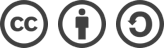## POJ 4047 Garden（线段树 - 区间增减、区间查询）解题报告

2016-09-30 1,612 次浏览

# 题面

Garden
Time Limit: 1000MS Memory Limit: 65536K

Description
There are n flowerpots in Daniel's garden. These flowerpots are placed in n positions, and these n positions are numbered from 1 to n. Each flower is assigned an aesthetic value. The aesthetic values vary during different time of a day and different seasons of a year. Naughty Daniel is a happy and hardworking gardener who takes pleasure in exchanging the position of the flowerpots.
Friends of Daniel are great fans of his miniature gardens. Before they visit Daniel's home, they will take their old-fashioned cameras which are unable to adjust the focus so that it can give a shot of exactly k consecutive flowerpots. Daniel hopes his friends enjoy themselves, but he doesn't want his friend to see all of his flowers due to some secret reasons, so he guides his friends to the best place to catch the most beautiful view in the interval [x, y], that is to say, to maximize the sum of the aesthetics values of the k flowerpots taken in one camera shot when they are only allow to see the flowerpots between position x to position y.
There are m operations or queries are given in form of (p, x, y), here p = 0, 1 or 2. The meanings of different value of p are shown below.

1. p=0 settheaestheticvalueofthepotinpositionxasy.(1<=x<=n;-100<= y <= 100)
2. p = 1 exchange the pot in position x and the pot in position y. (1 <= x, y <= n; x might equal to y)
3. p = 2 print the maximum sum of aesthetics values of one camera shot in interval [x, y]. (1 <= x <= y <= n; We guarantee that y-x+1>=k)

Input
There are multiple test cases.
The first line of the input file contains only one integer indicates the number of test cases.
For each test case, the first line contains three integers: n, m, k (1 <= k <= n <= 200,000; 0 <= m <= 200,000).
The second line contains n integers indicates the initial aesthetic values of flowers from position 1 to position n. Some flowers are sick, so their aesthetic values are negative integers. The aesthetic values range from -100 to 100. (Notice: The number of position is assigned 1 to n from left to right.)
In the next m lines, each line contains a triple (p, x, y). The meaning of triples is mentioned above.

Output
For each query with p = 2, print the maximum sum of the aesthetics values in oneshot in interval [x, y].

Sample Input
1
5 7 3
-1 2 -4 6 1
2 1 5
2 1 3
1 2 1
2 1 5
2 1 4
0 2 4
2 1 5

Sample Output
4
-3
3
1
6

# 参考代码

#include <cstdio>
#include <algorithm>
#define lson l, m, rt<<1
#define rson m+1, r, rt<<1|1
const int MAXN = 200001;
const int INF = 0x3f3f3f3f;
using namespace std;

int lazy[MAXN<<2];
int MAX[MAXN<<2];
int a[MAXN], sum[MAXN];

void PushUp(int rt) {
MAX[rt] = max(MAX[rt<<1], MAX[rt<<1|1]);
}

void PushDown(int rt) {
if(lazy[rt]) {
lazy[rt<<1] += lazy[rt];
lazy[rt<<1|1] += lazy[rt];
MAX[rt<<1] += lazy[rt];
MAX[rt<<1|1] += lazy[rt];
lazy[rt] = 0;
}
}

void Build(int l, int r, int rt) {
lazy[rt] = 0;
if (l == r) {
MAX[rt] = sum[l];
return;
}
int m = (l + r) >> 1;
Build(lson);
Build(rson);
PushUp(rt);
}

void Update(int L, int R, int v, int l, int r, int rt) {
if(L<=l && r<=R) {
lazy[rt] += v;
MAX[rt] += v;
return;
}
PushDown(rt);
int m = (l + r) >> 1;
if(L <= m) Update(L, R, v, lson);
if(m < R) Update(L, R, v, rson);
PushUp(rt);
}

int QueryMAX(int L, int R, int l, int r, int rt) {
if(L <= l && r <= R) {
return MAX[rt];
}
PushDown(rt);
int m = (l + r) >> 1;
int ret = -INF;
if(L <= m) ret = max(ret, QueryMAX(L, R, lson));
if(m < R) ret = max(ret, QueryMAX(L, R, rson));
return ret;
}

int main(int argc, char const *argv[]) {
int t, n, m, k, p, x, y;
scanf("%d", &t);
while(t--) {
scanf("%d %d %d", &n, &m, &k);
for(int i=1; i<=n; ++i) {
scanf("%d", &a[i]);
}
// 计算区间内元素和数组 sum
sum = 0;
for(int i=1; i<=k; ++i) {
sum += a[i];
}
for(int i=2; i<=n-k+1; ++i) {
sum[i] = sum[i-1]-a[i-1] + a[i+k-1];
}
// 重设 n 为 sum 数组的长度
n = n-k+1;
// 构造线段树
Build(1, n, 1);
while(m--) {
scanf("%d %d %d", &p, &x, &y);
if(p == 0) {
int d = y-a[x];
a[x] = y;
Update(max(1, x-k+1), x, d, 1, n, 1);
}
if(p == 1) { // 两次操作 0
int tmp = a[x];
int d = a[y]-a[x];
a[x] = a[y];
Update(max(1, x-k+1), x, d, 1, n, 1);
d = tmp-a[y];
a[y] = tmp;
Update(max(1, y-k+1), y, d, 1, n, 1);
}
if(p == 2)
printf("%d\n", QueryMAX(x, y-k+1, 1, n, 1));
}
}

return 0;
}bLue 创作，采用 知识共享署名 3.0，可自由转载、引用，但需署名作者且注明文章出处。